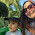## Tuesday, February 16, 2016

### An Easier Way To Do Long Division

Long division is not fun. At least according to my 11 year old.  However, there is an approach to make  it more accessible, especially for kids who look at the problem and have no idea where to start. Division is often taught to young kids as the process of partitioning an amount into smaller groups. We give them ten Lego guys and ask them how many groups of two they can make. As kids get a little older, we point out that division is the inverse of multiplication, which usually works well with facts. But if multiplication is just repeated addition, wouldn't the inverse of that be repeated subtraction? Yes! Viewing division as repeated subtraction can remove the deer in the headlights stare we often see when a child faces a long division problem. Let's start with a simple example:

If we start with 10÷5, we can subtract 5 once from 10, leaving us with 5, then subtract 5 once again and we have 0. We subtracted 2 fives, so the answer is 2. Follow?

Now for bigger problems. Kids are usually comfortable with doubling a number or multiplying it by 10. Multiplying by 5 is just halving ten, so that's not usually a problem, either. We will work with multiplying the divisor by 1, 2, 5, or 10, using repeated subtraction, to solve the problem. That's it. Let's look at my super high tech example:

The numbers on the right side indicate whether I multiplied the divisor by 1, 2, 5 or 10.  Once I get to 0 (or less than the divisor), I add the numbers on the right to get my answer. Note that you are always subtracting from the whole dividend, not portions of it as in the standard algorithm. Here's another example:

When kids get comfortable with this method, they start seeing that doubling the double is times 4, or if times ten is slightly too big, they will subtract one divisor to get times 9, and that's exactly the reasoning we are looking for! Conversely, some kids are only able to start with doubling and multiplying by ten, and that's okay, too. The problem may take awhile to finish, but the calculations are easy, which reduces anxiety.

I did show my kids the standard approach later, after they had mastered this one, and allowed them to choose what made the most sense to them. They both thought this way was easier, and allowed them to tackle the problem right away with confidence. Once your kids understand the concept of long division and can accurately solve problems, do them a favor and move on. Calculation is a small portion of mathematics, and in reality, nobody does long division by hand. Of course you and I will grab a calculator, but even people who use math in their careers every day use a computer or calculator to do computations. Math is reasoning, patterns, problem solving, spatial thinking; get to the good stuff once they have basic competency and stop beating a dead horse with pages of long division. Here's a link to my periscope about this strategy.

1.2.What if You Cant Subtract It When You add The Extra Zeros? Then What Do You Subtract With??

1.Because I Tried And I Couldn't Subtract Because The Bigger Number Was On The Bottom

2.Because I Tried And I Couldn't Subtract Because The Bigger Number Was On The Bottom

3.You shouldn't be adding any extra zeros. You use the whole number dividend, not part of it as in standard method.